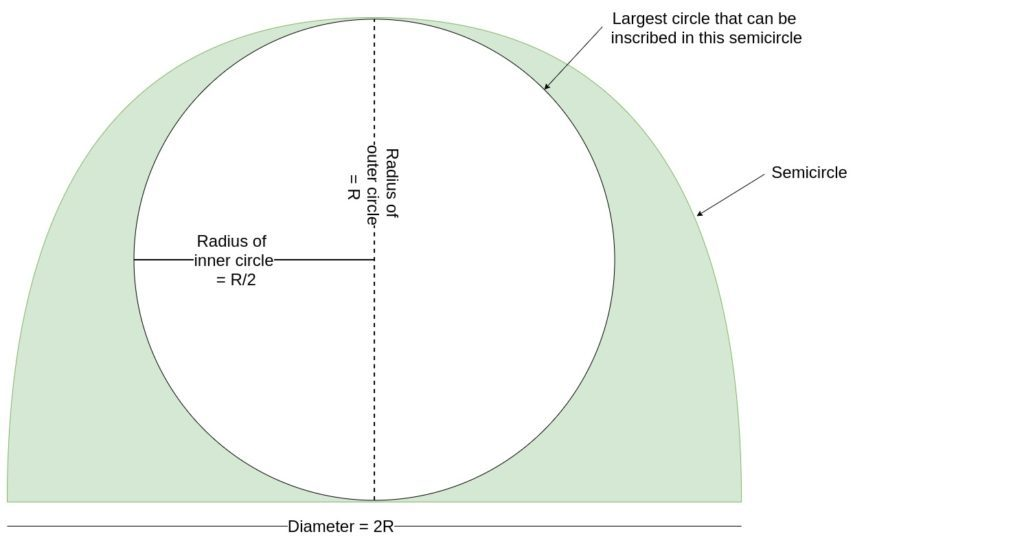# Area of largest Circle that can be inscribed in a SemiCircle

• Last Updated : 21 Jun, 2022

Given a semicircle with radius R, the task is to find the area of the largest circle that can be inscribed in the semicircle.
Examples:

```Input: R = 2
Output: 3.14

Input: R = 8
Output: 50.24```

Approach: Let R be the radius of the semicircle

1. For Largest circle that can be inscribed in this semicircle, the diameter of the circle must be equal to the radius of the semi-circle.

2. So, if the radius of the semi-circle is R, then the diameter of the largest inscribed circle will be R.
3. Hence the radius of the inscribed circle must be R/2
4. Therefore the area of the largest circle will be

```Area of circle = pi*Radius2
= pi*(R/2)2

since the radius of largest circle is R/2
where R is the radius of the semicircle```
1.Below is the implementation of the above approach:

## C++

 `// C++ Program to find the biggest circle``// which can be inscribed within the semicircle` `#include ``using` `namespace` `std;` `// Function to find the area``// of the circle``float` `circlearea(``float` `R)``{` `    ``// Radius cannot be negative``    ``if` `(R < 0)``        ``return` `-1;` `    ``// Area of the largest circle``    ``float` `a = 3.14 * R * R / 4;` `    ``return` `a;``}` `// Driver code``int` `main()``{``    ``float` `R = 2;``    ``cout << circlearea(R) << endl;` `    ``return` `0;``}`

## Java

 `// Java Program to find the biggest circle``// which can be inscribed within the semicircle``class` `GFG``{``    ` `    ``// Function to find the area``    ``// of the circle``    ``static` `float` `circlearea(``float` `R)``    ``{``    ` `        ``// Radius cannot be negative``        ``if` `(R < ``0``)``            ``return` `-``1``;``    ` `        ``// Area of the largest circle``        ``float` `a = (``float``)((``3.14` `* R * R) / ``4``);``    ` `        ``return` `a;``    ``}``    ` `    ``// Driver code``    ``public` `static` `void` `main (String[] args)``    ``{``        ``float` `R = ``2``;``        ``System.out.println(circlearea(R));``    ``}``}` `// This code is contributed by AnkitRai01`

## Python3

 `# Python3 Program to find the biggest circle``# which can be inscribed within the semicircle` `# Function to find the area``# of the circle``def` `circlearea(R) :` `    ``# Radius cannot be negative``    ``if` `(R < ``0``) :``        ``return` `-``1``;` `    ``# Area of the largest circle``    ``a ``=` `(``3.14` `*` `R ``*` `R) ``/` `4``;` `    ``return` `a;` `# Driver code``if` `__name__ ``=``=` `"__main__"` `:` `    ``R ``=` `2``;``    ``print``(circlearea(R)) ;``    ` `# This code is contributed by AnkitRai01`

## C#

 `// C# Program to find the biggest circle``// which can be inscribed within the semicircle``using` `System;` `class` `GFG``{``    ` `    ``// Function to find the area``    ``// of the circle``    ``static` `float` `circlearea(``float` `R)``    ``{``    ` `        ``// Radius cannot be negative``        ``if` `(R < 0)``            ``return` `-1;``    ` `        ``// Area of the largest circle``        ``float` `a = (``float``)((3.14 * R * R) / 4);``    ` `        ``return` `a;``    ``}``    ` `    ``// Driver code``    ``public` `static` `void` `Main (``string``[] args)``    ``{``        ``float` `R = 2;``        ``Console.WriteLine(circlearea(R));``    ``}``}` `// This code is contributed by AnkitRai01`

## Javascript

 ``

Output:

`3.14`

Time Complexity: O(1)

Auxiliary Space: O(1)

My Personal Notes arrow_drop_up